# PSAT Math : How to find the ratio of a fraction

## Example Questions

### Example Question #49 : Proportion / Ratio / Rate

A TV show lasts 30 minutes, what fraction of the show is left after 12 minutes have passed?

12/15

1/3

3/5

2/3

4/10

3/5

Explanation:

After watching 12 minutes of the show 18 remain. 18 is 60% of the total 30 minutes. As a fraction it can be expressed as 3/5.

### Example Question #50 : Proportion / Ratio / Rate

A bag contains red, orange, and yellow marbles only. The marbles occur in a ratio of 5 red marbles: 4 orange marbles: 1 yellow marble. If one-third of the red marbles, one-half of the orange marbles, and one-fourth of the yellow marbles are removed, then what fraction of the remaining marbles in the bag is red?

39/73

43/75

40/73

40/43

37/77

40/73

Explanation: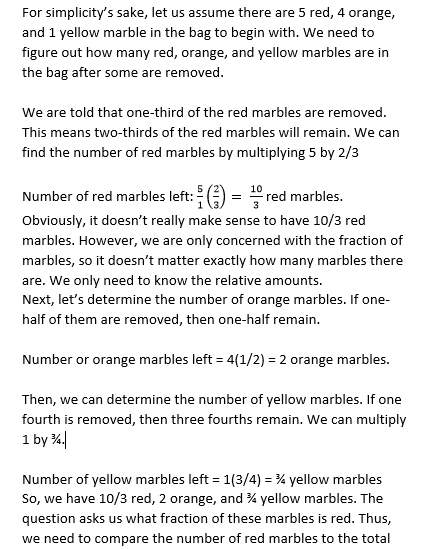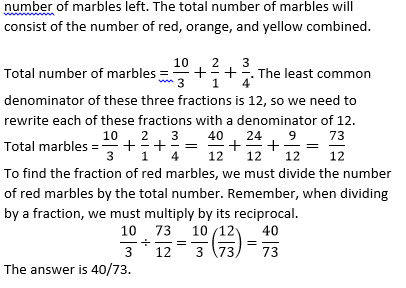### Example Question #1 : How To Find The Ratio Of A Fraction

Marty drove 40 mi/hr for 3 hours, then 60 mi/hr for 1 hour, and finally 70 mi/hr for the last 2 hours. What was Marty's average speed?

53 mi/hr

60 mi/hr

47 mi/hr

58 mi/hr

63 mi/hr

53 mi/hr

Explanation:

Marty's total driving time was 3 + 1 + 2 = 6 hours. He drove 40 mi/hr for 3 hours, or 3/6 = 1/2 of the time. He drove 60 mi/hr for 1 hour, or 1/6 of the drive. Lastly, he drove 70 mi/hr for 2 hours, or 2/6 = 1/3 of the drive.

To find the average speed, we need to multiply the speeds with their corresponding weights and add them up.

Average = 1/2 * 40 + 1/6 * 60 + 1/3 * 70 = 53.33... ≈ 53 mi/hr

### Example Question #1 : How To Find The Ratio Of A Fraction

A deck of cards has 52 cards. What is the probability of dealing a card with a face value of 4?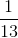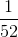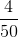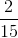Explanation:

For this problem we need to recall that that there are 4 different suits in a deck of cards. Each suit has numbers 1 through 10, jack, queen, king, and ace. Therefore, we can write a ratio of number of 4's over total cards in the deck.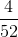From here we can reduce the fraction and we get### Example Question #2 : How To Find The Ratio Of A Fraction

There is a 5 to 3 ratio of red marbles to green marbles in a bucket. How many red marbles can be in a bucket of 40 marbles?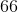Explanation:

In this problem, we use a proportion to solve for the number of red marbles in the bucket. Since the ratio is 5 to 3 we can set up a proportion of the red over total where the total is (red marbles  + green marbles). We then set this proportion equal toover our new total, which is 40. Then we cross multiply and divide to get the number of red marbles in a bucket of 40 total marbles.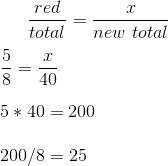### Example Question #3 : How To Find The Ratio Of A Fraction

There is a 2 to 3 ratio of dogs to cats in a park. How many cats are there if there are 12 dogs?Explanation:

We set up the following ratio of dogs to cats and set it equal to the new ratio we are interested in.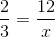Then we cross multiply and divide to solve for.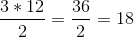### Example Question #4 : How To Find The Ratio Of A Fraction

You have a bag filled with red and green apples. If there are 9 red apples and 12 green apples, what is the probability of choosing a red apple out of the bag?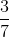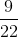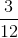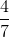Explanation:

To find the probability we need to set up a ratio. The ratio will be how many red apples are in the bag over the total number of apples (both red and green) in the bag.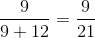We can now reduce this fraction: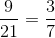### Example Question #3 : How To Find The Ratio Of A Fraction

A pie is made up ofcrust,apples, andsugar, and the rest is jelly. What is the ratio of crust to jelly?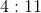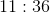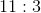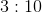Explanation:

A pie is made up ofcrust,apples,sugar, and the rest is jelly. What is the ratio of crust to jelly?

To compute this ratio, you must first ascertain how much of the pie is jelly. This is: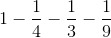Begin by using the common denominator: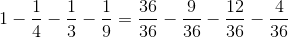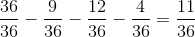So, the ratio of crust to jelly is: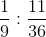This can be written as the fraction: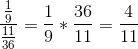, or### Example Question #4 : How To Find The Ratio Of A Fraction

In a solution,of the fluid is water,is wine, and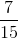is lemon juice. What is the ratio of lemon juice to water?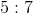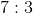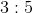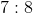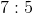Explanation:

This problem is really an easy fraction division. You should first divide the lemon juice amount by the water amount: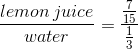Remember, to divide fractions, you multiply by the reciprocal: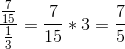This is the same as saying:### Example Question #5 : How To Find The Ratio Of A Fraction

If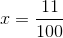and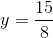, what is the ratio ofto?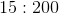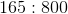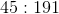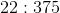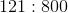Explanation:

To find a ratio like this, you simply need to make the fraction that represents the division of the two values by each other. Therefore, we have: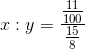Recall that division of fractions requires you to multiply by the reciprocal: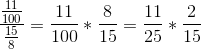which is the same as: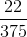This is the same as the ratio: